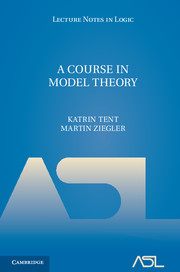# Lecture Notes in Logic, 40

## A Course in Model Theory

Katrin Tent, Martin ZieglerYear: 2012

ISBN-13: 9780521763240
258 pages. Hardcover.
Buy now (when ordering, include the discount code ASL2016 to receive the 25% ASL member discount)

This concise introduction to model theory begins with standard notions and takes the reader through to more advanced topics such as stability, simplicity and Hrushovski constructions. The authors introduce the classic results, as well as more recent developments in this vibrant area of mathematical logic. Concrete mathematical examples are included throughout to make the concepts easier to follow. The book also contains over 200 exercises, many with solutions, making the book a useful resource for graduate students as well as researchers.

1. The basics
2. Elementary extensions and compactness
3. Quantifier elimination
4. Countable models
5. Aleph-1-categorical theories
6. Morley rank
7. Simple theories
8. Stable theories
9. Prime extensions
10. The fine structure of 1-categorical theories
1. Set theory
2. Fields
3. Combinatorics
4. Solutions of exercises